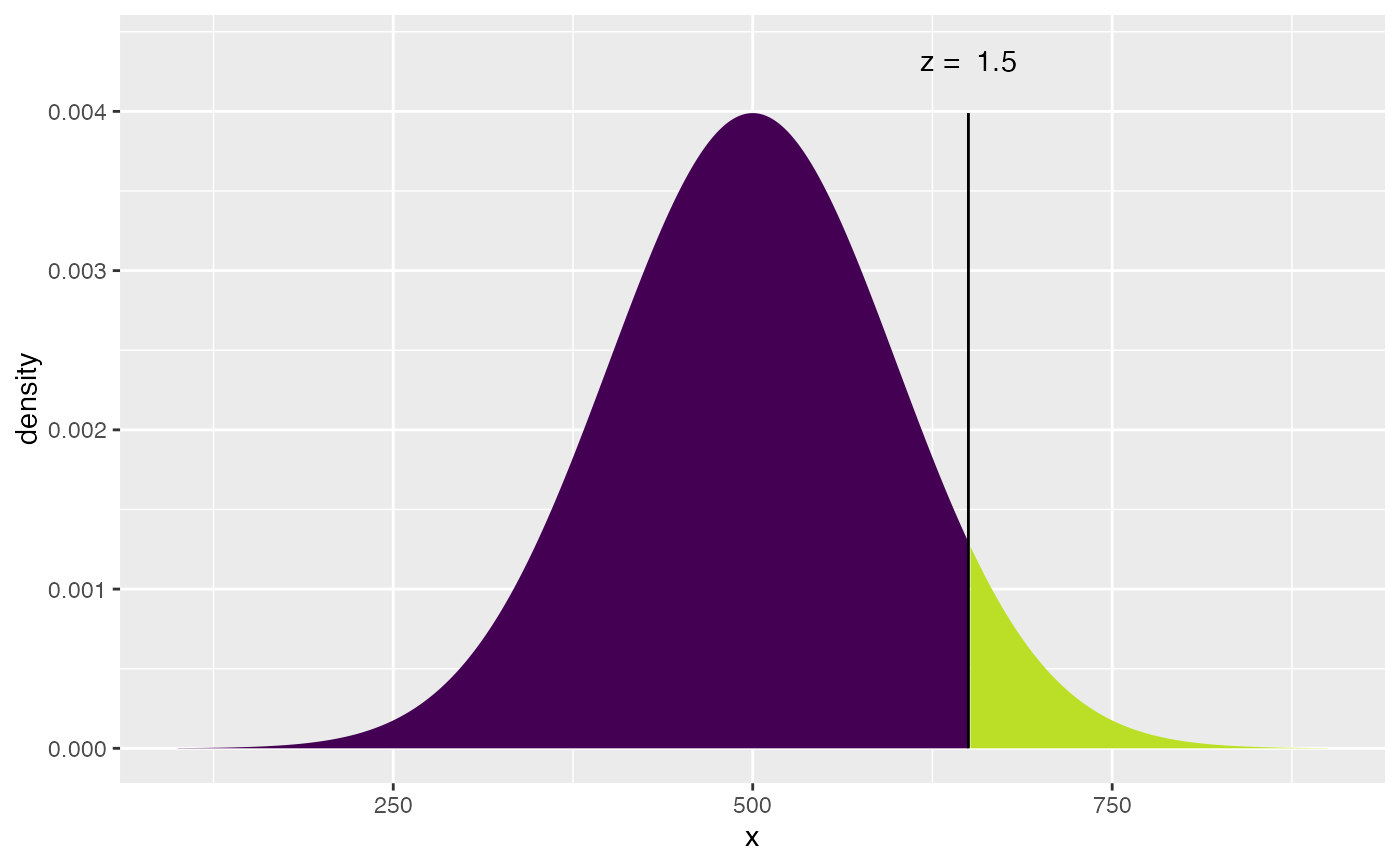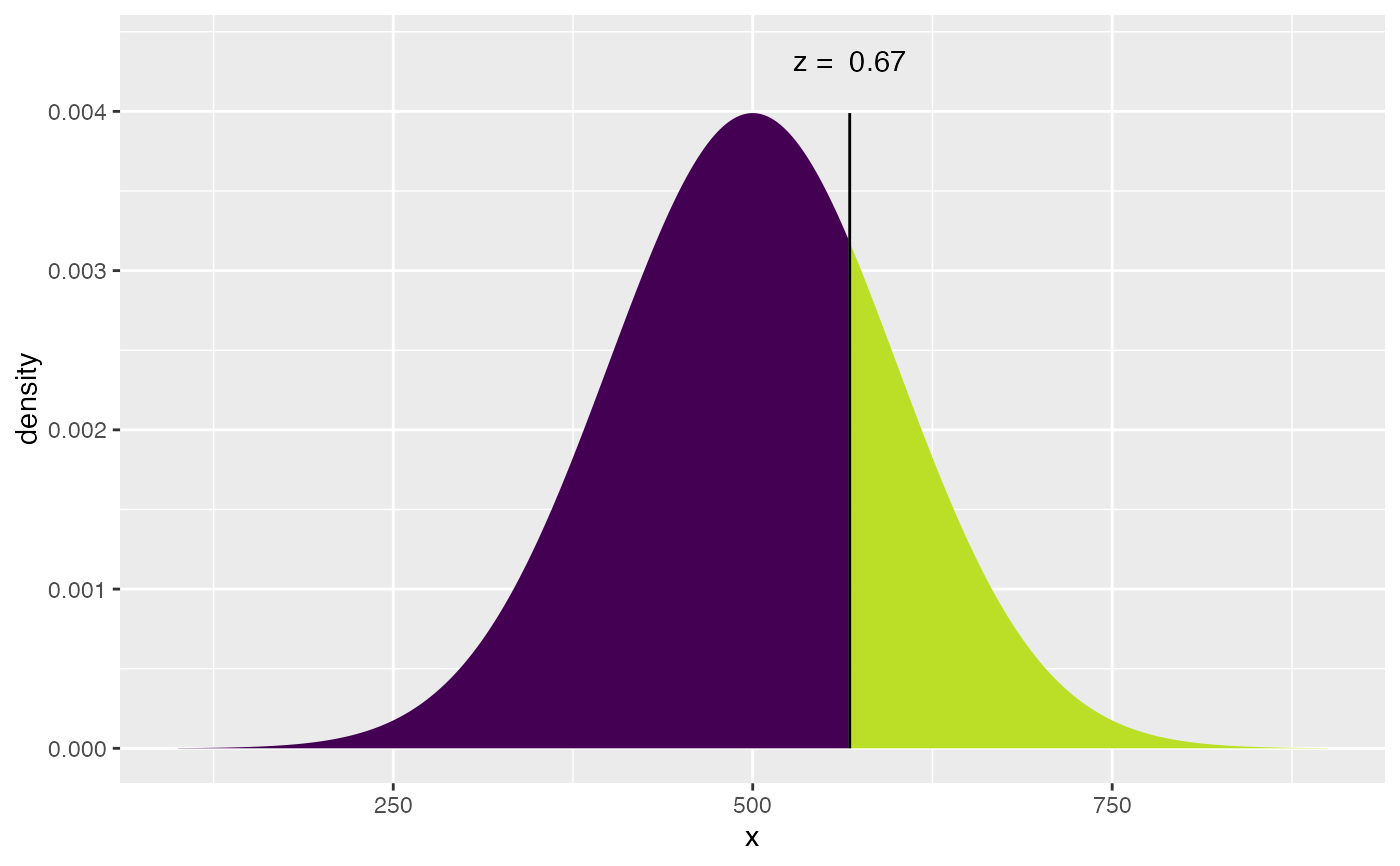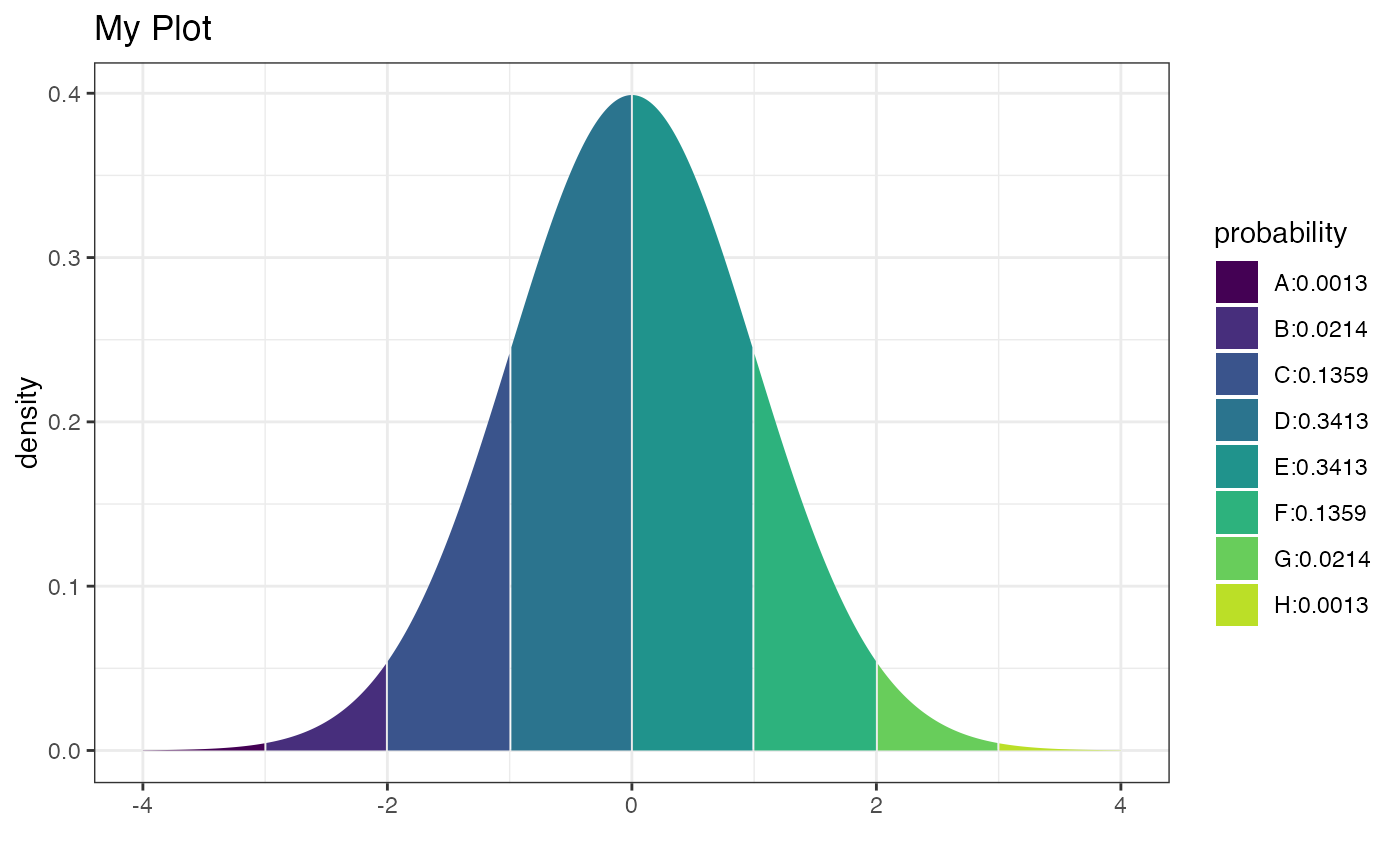These functions behave similarly to the functions with the initial x removed from their names but add more verbose output and graphics.

xpnorm(
q,
mean = 0,
sd = 1,
plot = TRUE,
verbose = TRUE,
invisible = FALSE,
digits = 4,
lower.tail = TRUE,
log.p = FALSE,
xlim = mean + c(-4, 4) * sd,
ylim = c(0, 1.4 * dnorm(mean, mean, sd)),
manipulate = FALSE,
...,
return = c("value", "plot")
)

xqnorm(
p,
mean = 0,
sd = 1,
plot = TRUE,
verbose = TRUE,
digits = getOption("digits"),
lower.tail = TRUE,
log.p = FALSE,
xlim,
ylim,
invisible = FALSE,
...,
return = c("value", "plot"),
pattern = c("stripes", "rings")
)

xcnorm(
p,
mean = 0,
sd = 1,
plot = TRUE,
verbose = TRUE,
digits = getOption("digits"),
lower.tail = TRUE,
log.p = FALSE,
xlim,
ylim,
invisible = FALSE,
...,
return = c("value", "plot"),
pattern = "rings"
)

## Arguments

q

quantile

mean, sd

parameters of normal distribution.

plot

logical. If TRUE, show an illustrative plot.

verbose

logical. If TRUE, display verbose output.

invisible

logical. If TRUE, return value invisibly.

digits

number of digits to display in output.

lower.tail

logical. If FALSE, use upper tail probabilities.

log.p

logical. If TRUE, uses the log of probabilities.

xlim, ylim

limits for plotting.

manipulate

logical. If TRUE and in RStudio, then sliders are added for interactivity.

...

return

If "plot", return a plot. If "values", return a vector of numerical values.

p

probability

pattern

One of "stripes" or "rings". In the latter case, pairs of regions (from inside to outside) are grouped together for coloring and probability calculation.

histogram(), chisq.test(), pnorm(), qnorm(), qqmath(), and plot().

## Examples

xpnorm(650, 500, 100)
#>
#> If X ~ N(500, 100), then
#> 	P(X <= 650) = P(Z <= 1.5) = 0.9332
#> 	P(X >  650) = P(Z >  1.5) = 0.06681
#>#>  0.9331928
xqnorm(.75, 500, 100)
#>
#> If X ~ N(500, 100), then
#> 	P(X <= 567.449) = 0.75
#> 	P(X >  567.449) = 0.25
#>#>  567.449
xpnorm(-3:3, return = "plot", system = "gg") %>%
gf_labs(title = "My Plot", x = "") %>%
gf_theme(theme_bw())
#>
#> If X ~ N(0, 1), then
#> 	P(X <= -3) = P(Z <= -3) = 0.00135	P(X <= -2) = P(Z <= -2) = 0.02275	P(X <= -1) = P(Z <= -1) = 0.15866	P(X <=  0) = P(Z <=  0) = 0.50000	P(X <=  1) = P(Z <=  1) = 0.84134	P(X <=  2) = P(Z <=  2) = 0.97725	P(X <=  3) = P(Z <=  3) = 0.99865
#> 	P(X >  -3) = P(Z >  -3) = 0.99865	P(X >  -2) = P(Z >  -2) = 0.97725	P(X >  -1) = P(Z >  -1) = 0.84134	P(X >   0) = P(Z >   0) = 0.50000	P(X >   1) = P(Z >   1) = 0.15866	P(X >   2) = P(Z >   2) = 0.02275	P(X >   3) = P(Z >   3) = 0.00135
#>if (FALSE) {
if (rstudio_is_available() & require(manipulate)) {
manipulate(xpnorm(score, 500, 100, verbose = verbose),
score = slider(200, 800),
verbose = checkbox(TRUE, label = "Verbose Output")
)
}
}